# Intermediate Maths Solutions for The Straight Line Exercise 3(b)

The Straight Line Exercise 3(b) Maths 1b textbook Chapter 3 Inter Solutions are given.

All problems with answers are given. These are very simple to understand.

Observe the example problems and solutions in the textbook.

Observe the given solutions and try them in your own method.

The Straight Line

Exercise 3(a)

SSC Maths text book Solutions class 10

Inter Maths 1A solutions

Inter Maths 1A textbook solutions

Inter Maths 1B solutions

Inter Maths 1IA text book solutions

Inter Maths IIB text book solutions

M

# Inter 1b Chapter 3 The Straight Line Exercise 3(b) Solutions

Chapter 3 – The Straight Line

Exercise 3(b)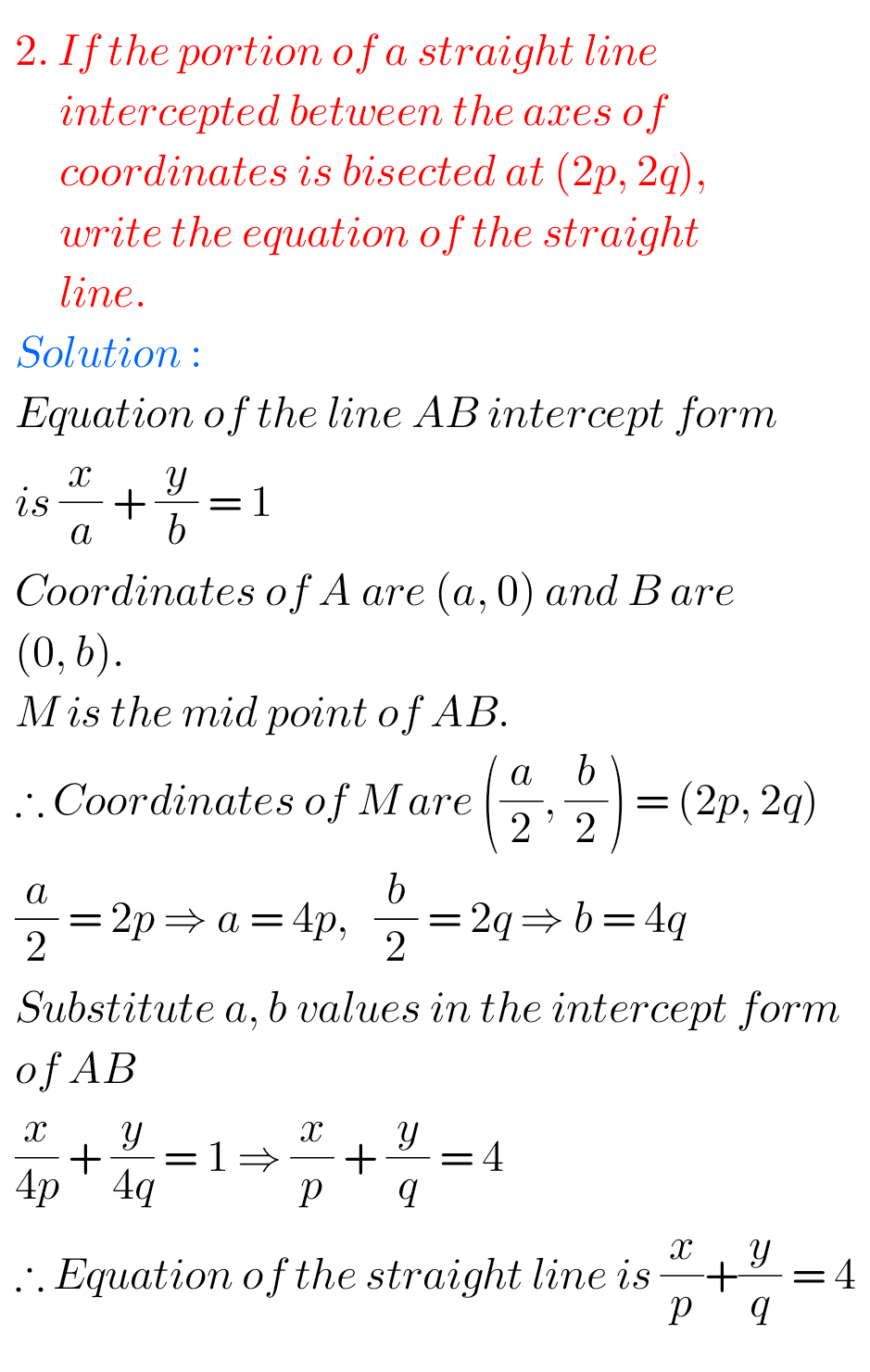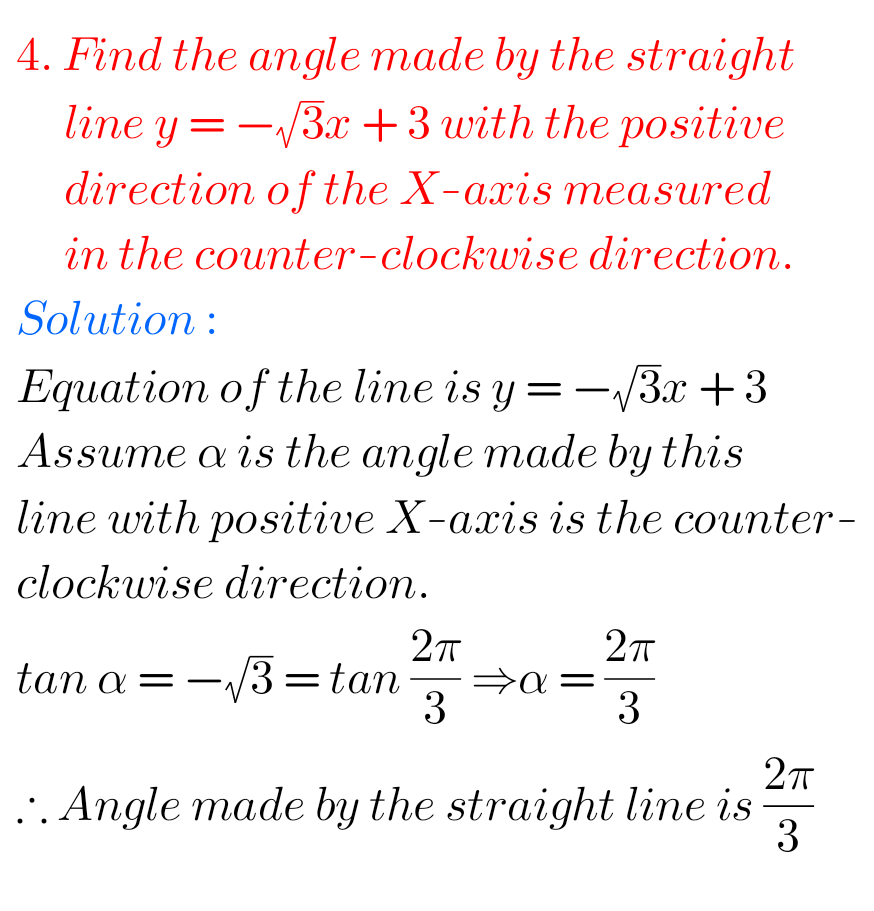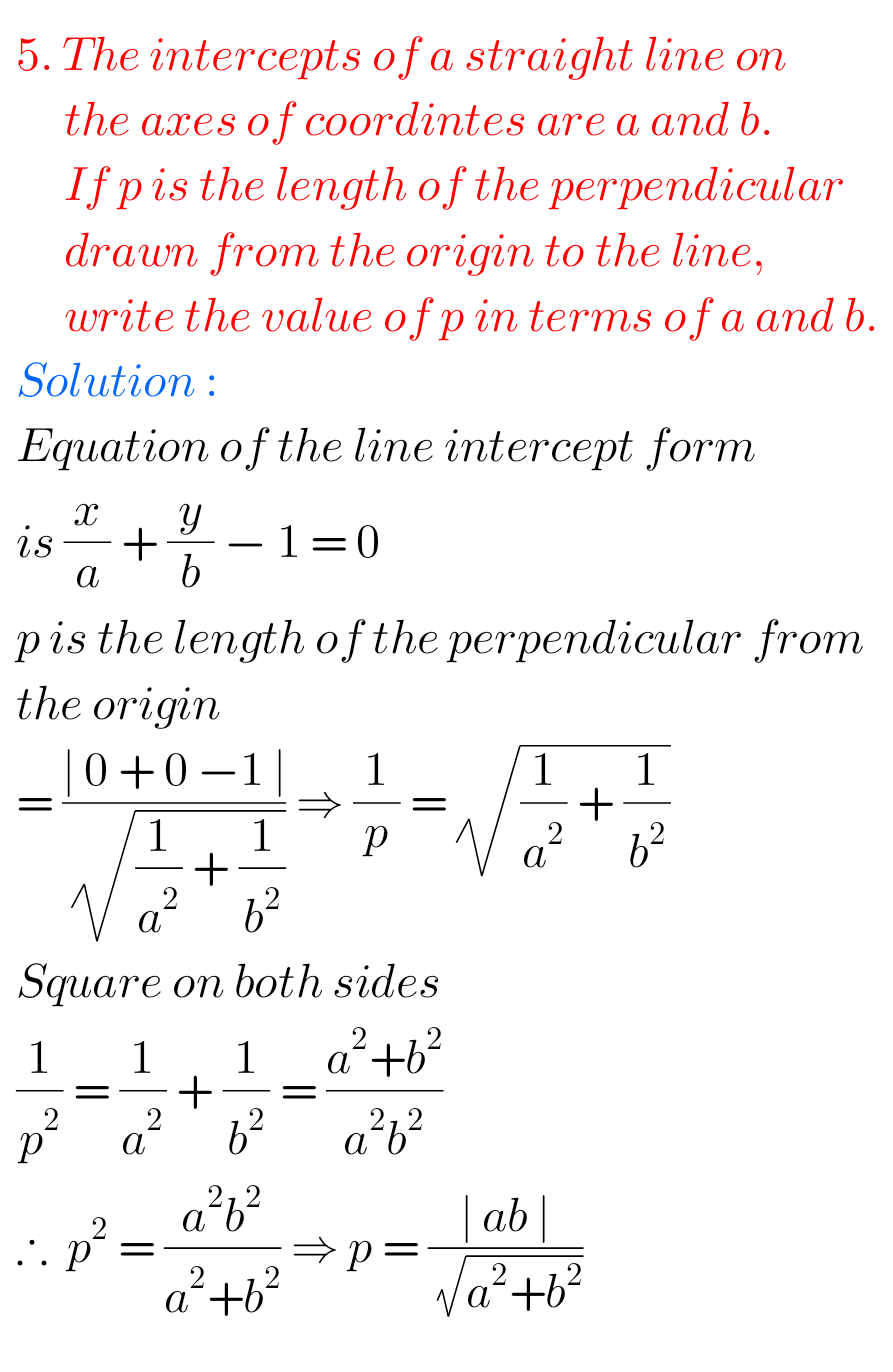## The Straight Line Exercise 3(b) Solutions Inter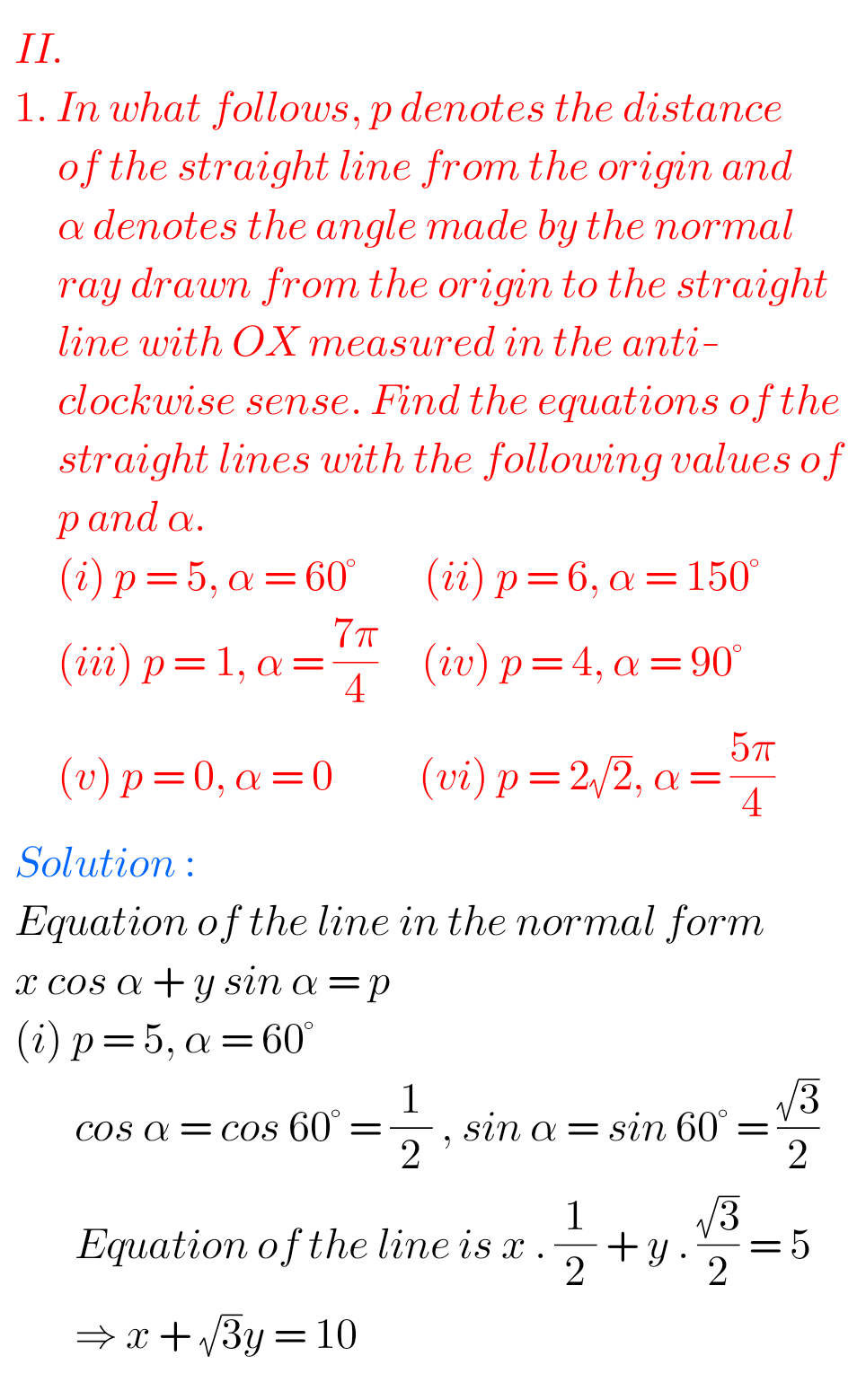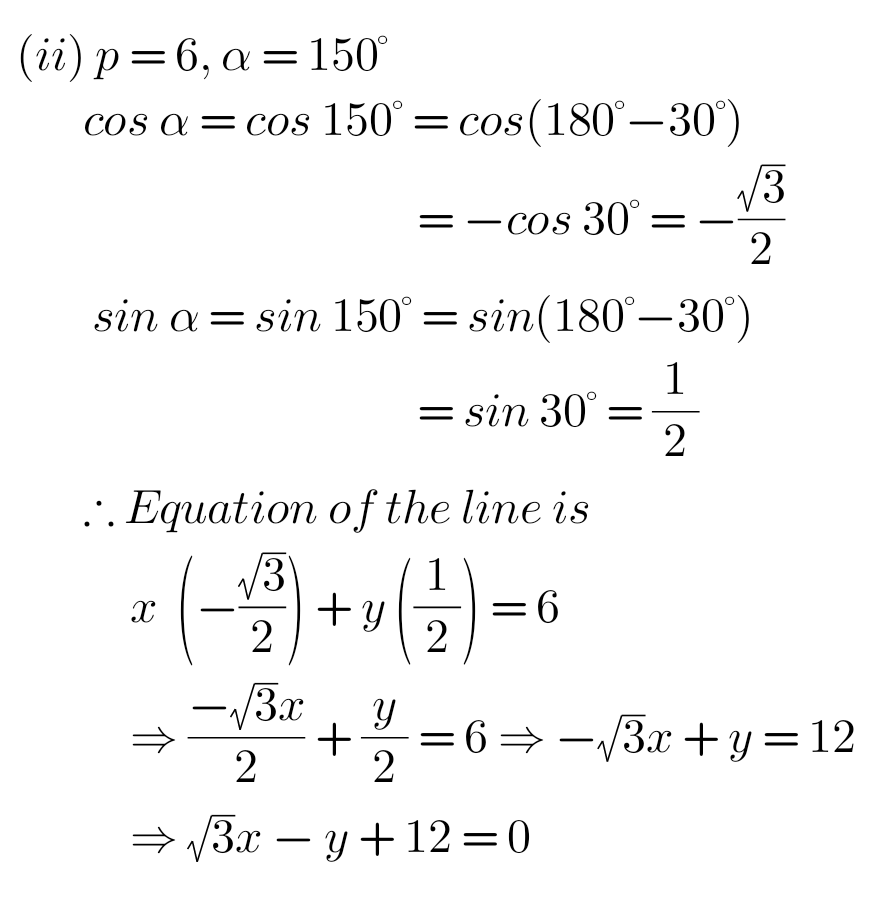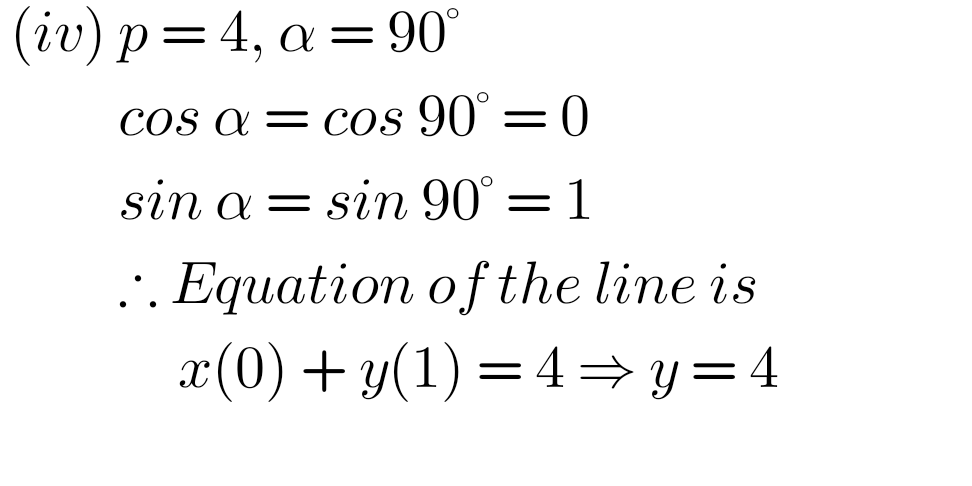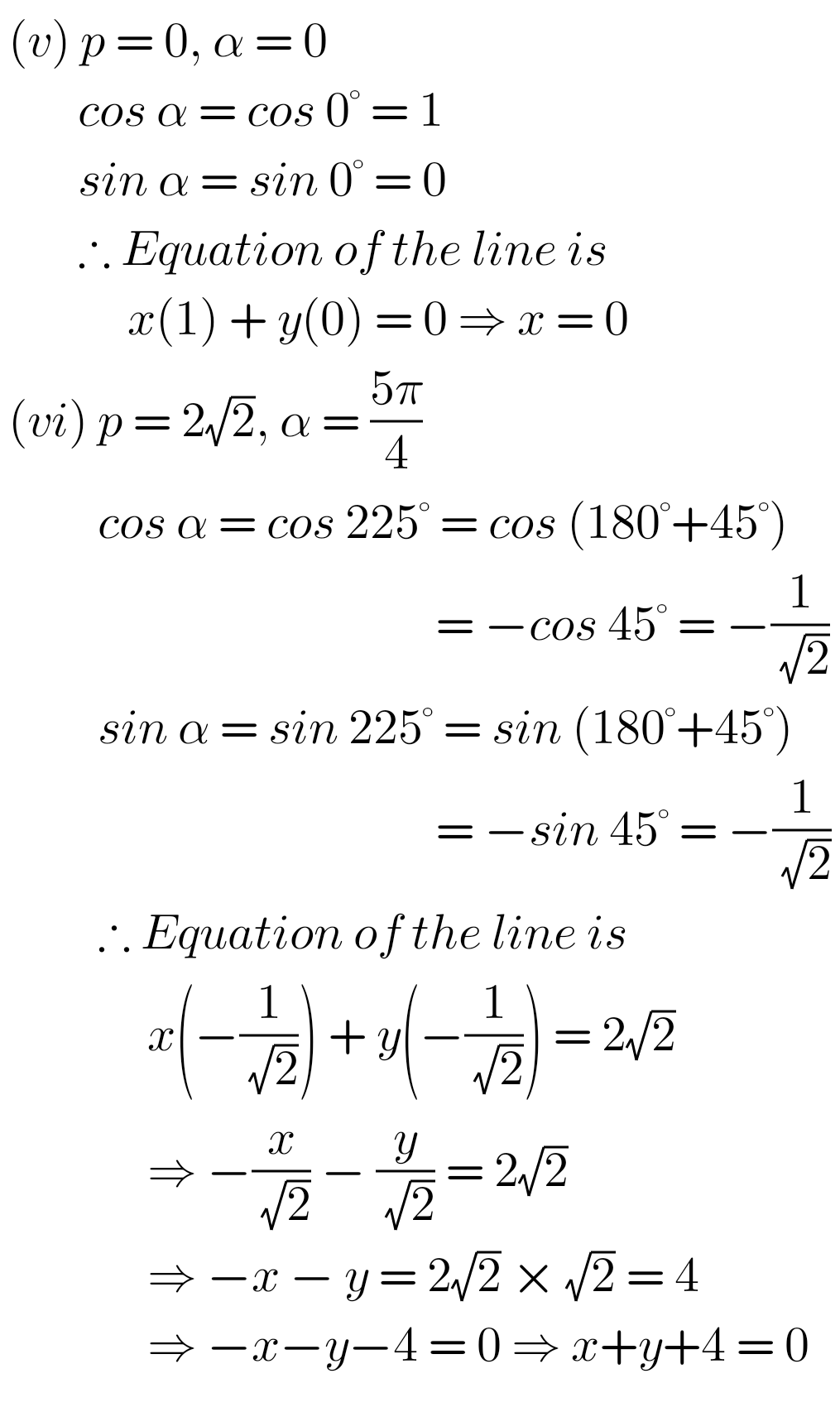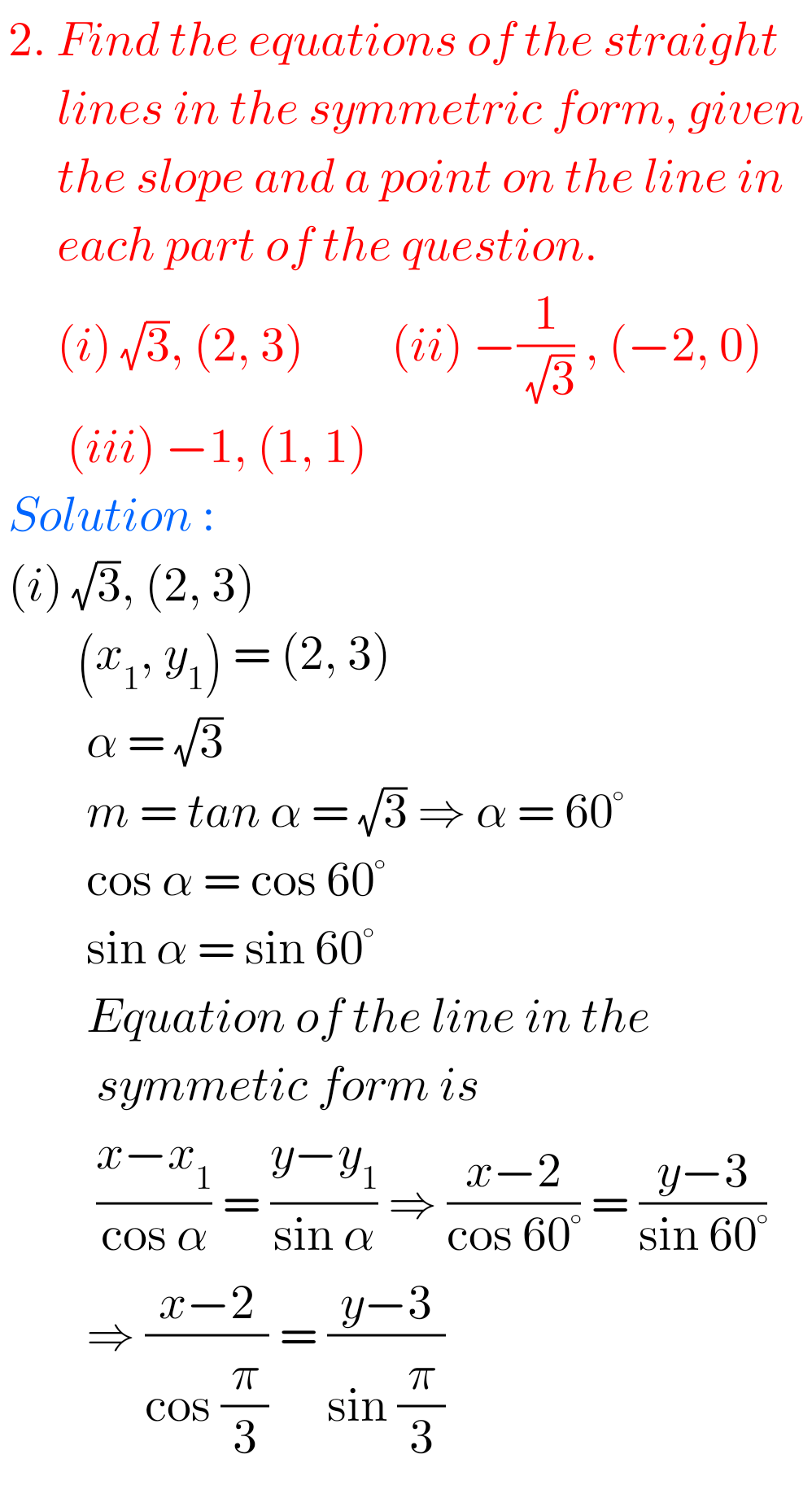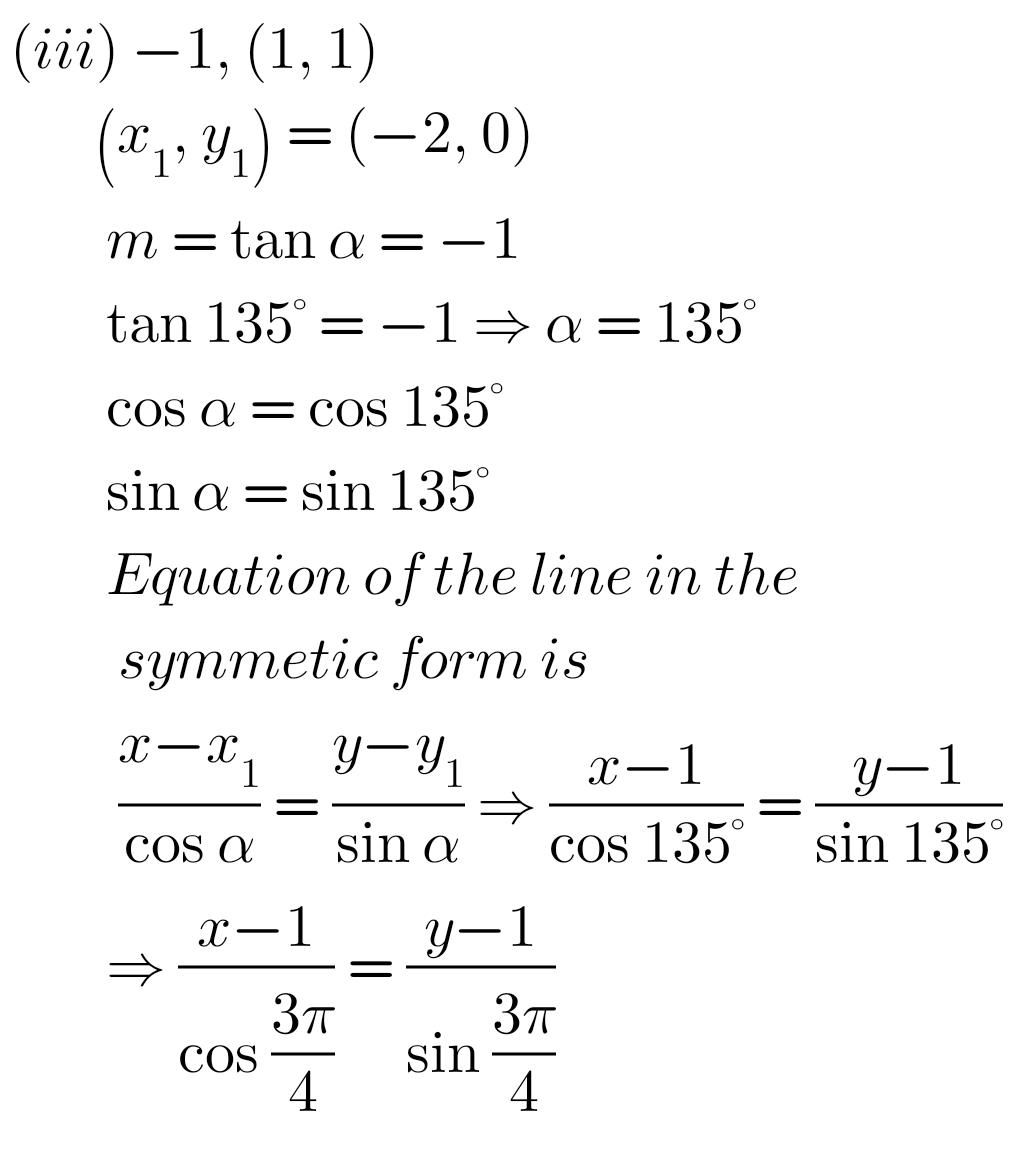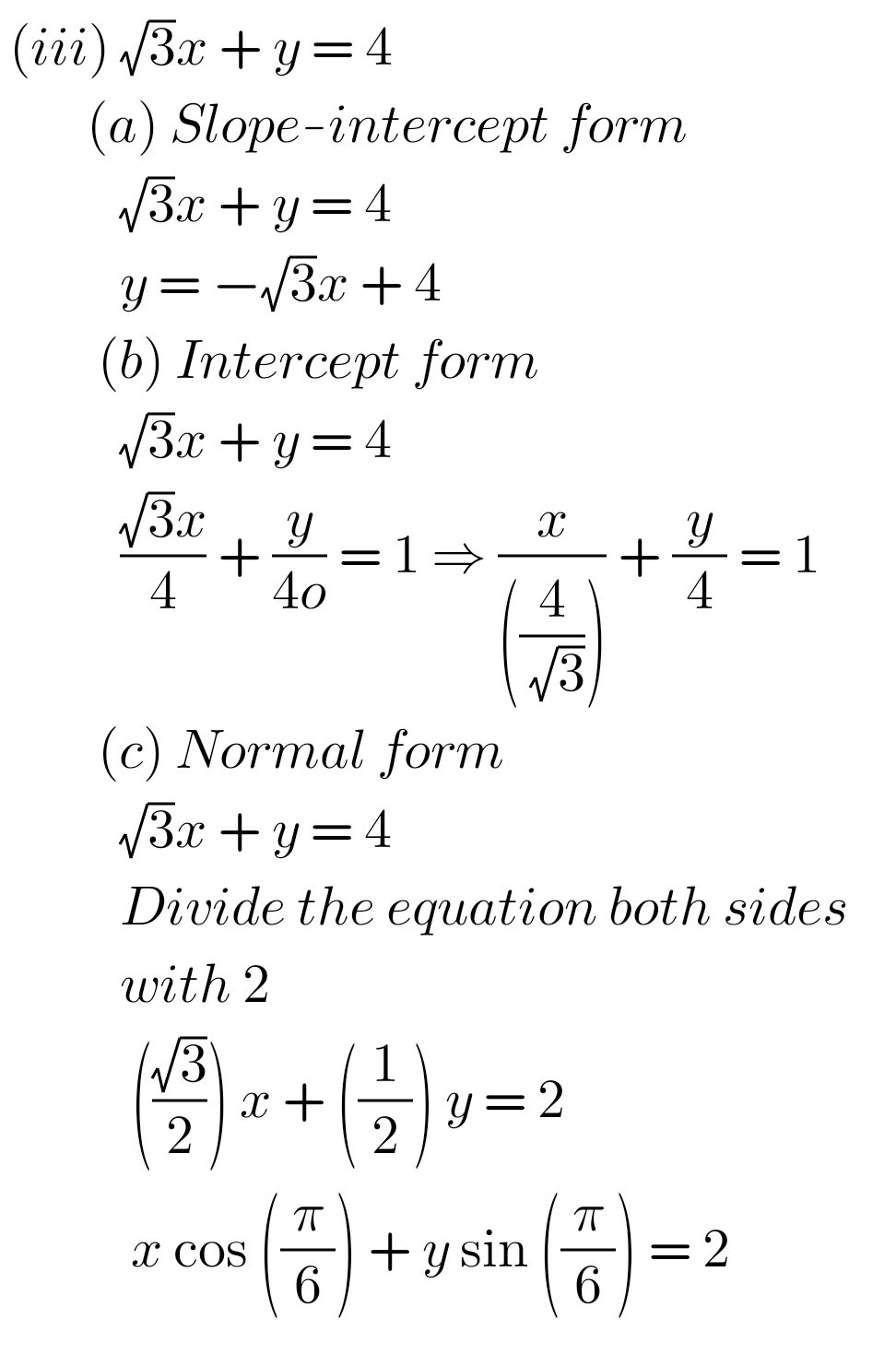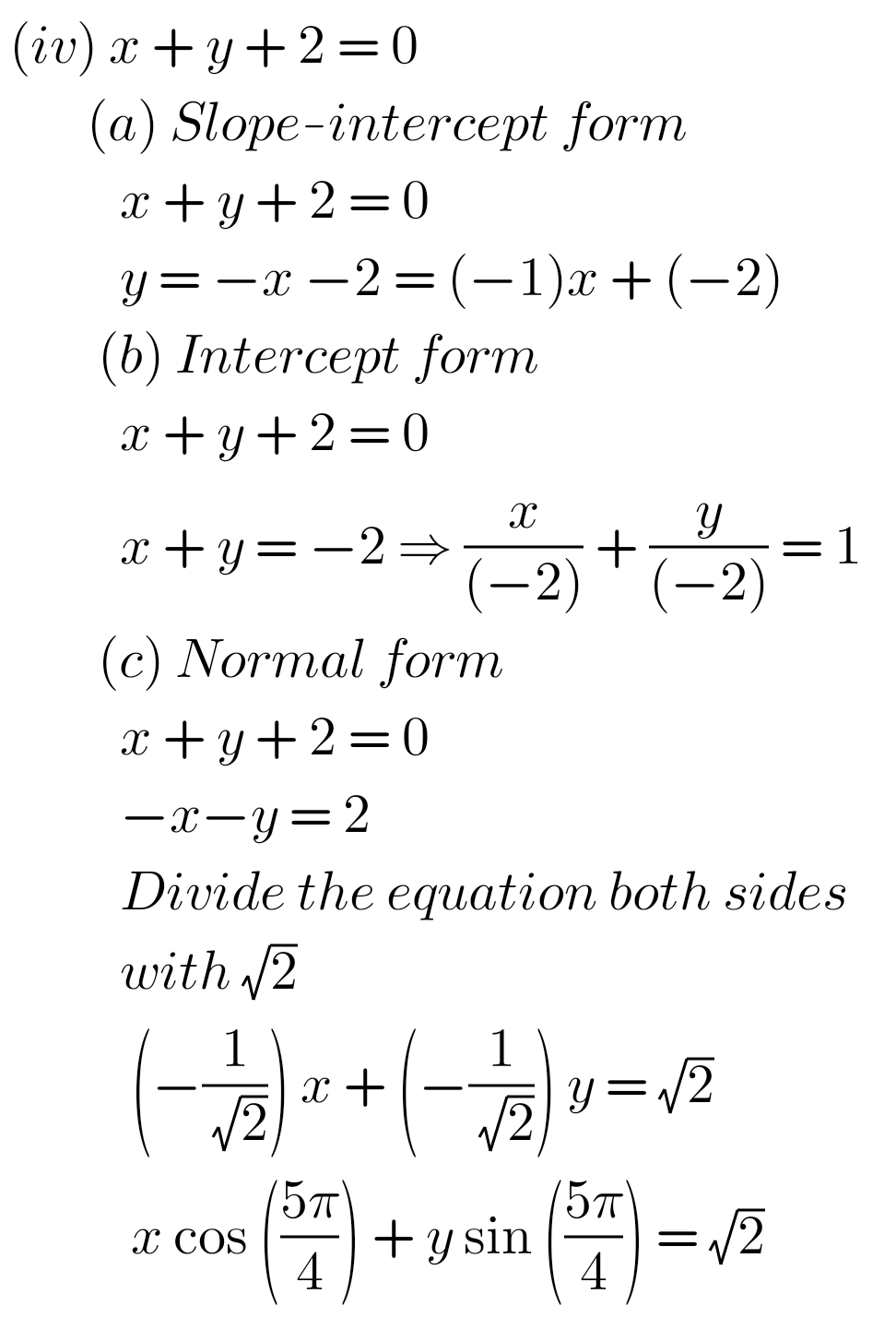##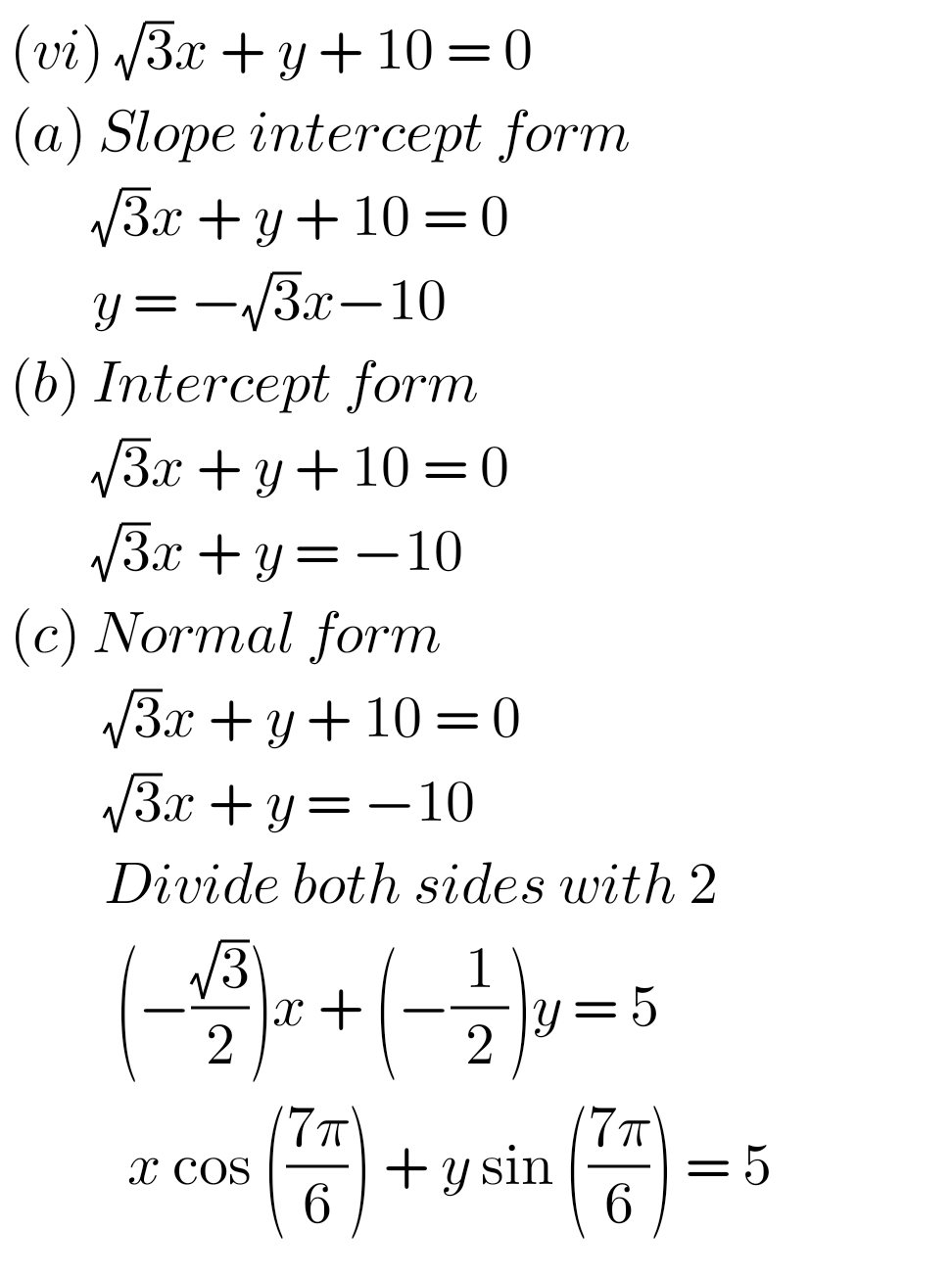## Solutions for Exercise 3(b) The Straight Line Inter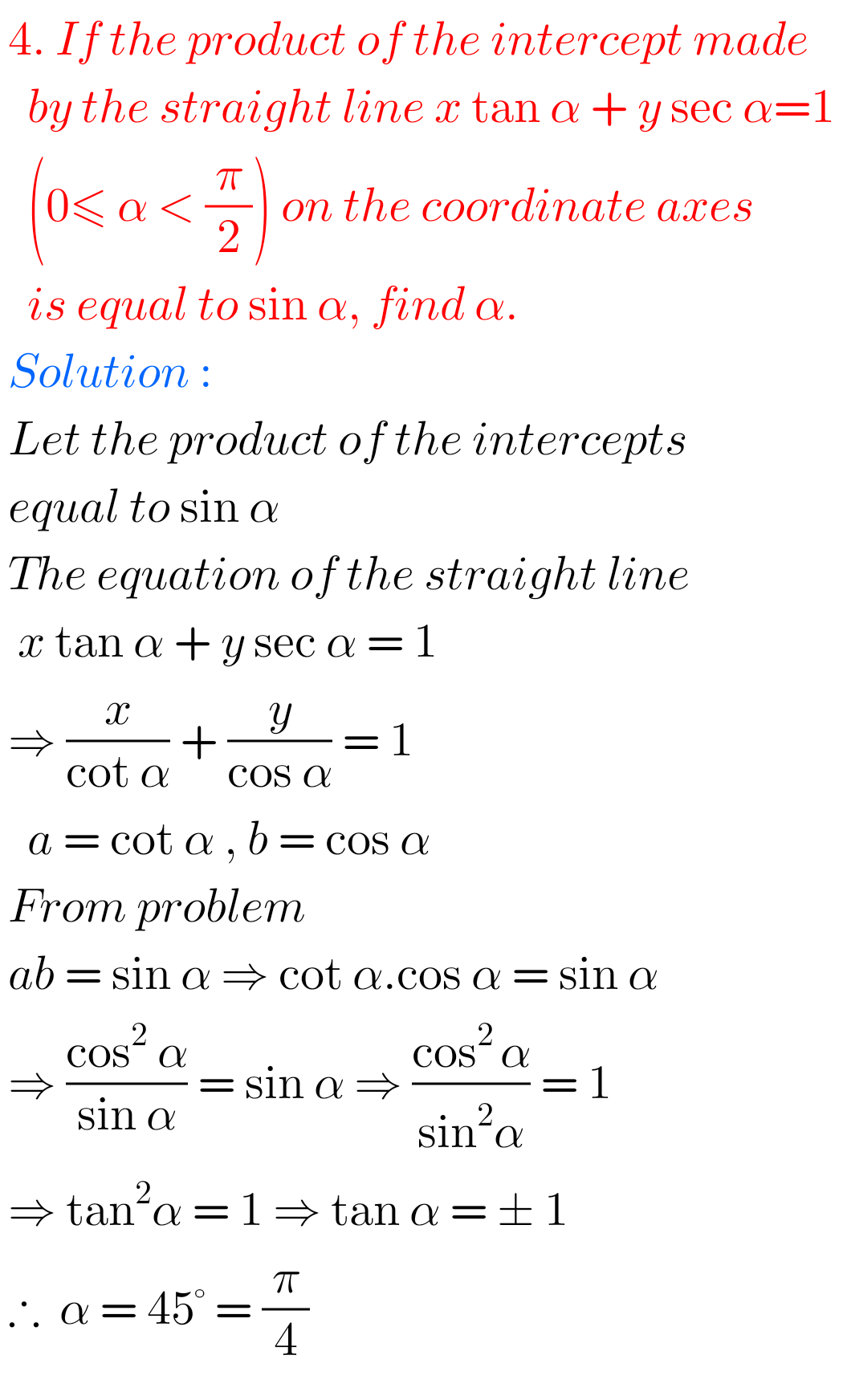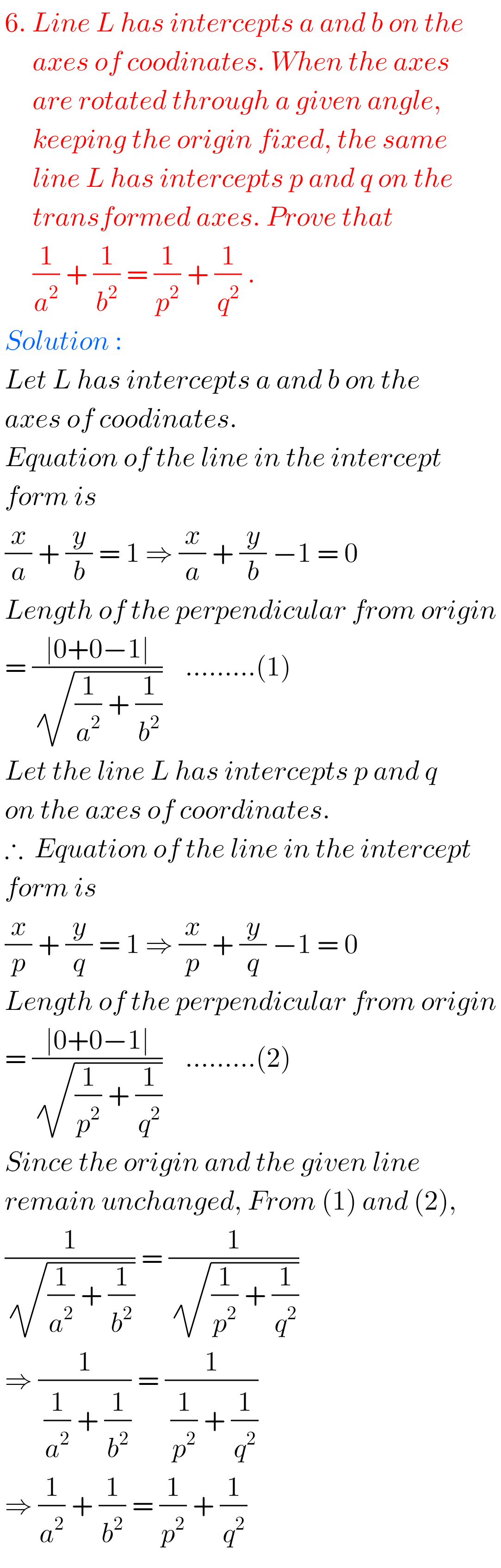### Maths Solutions for Exercise 3(b) The Straight Line Inter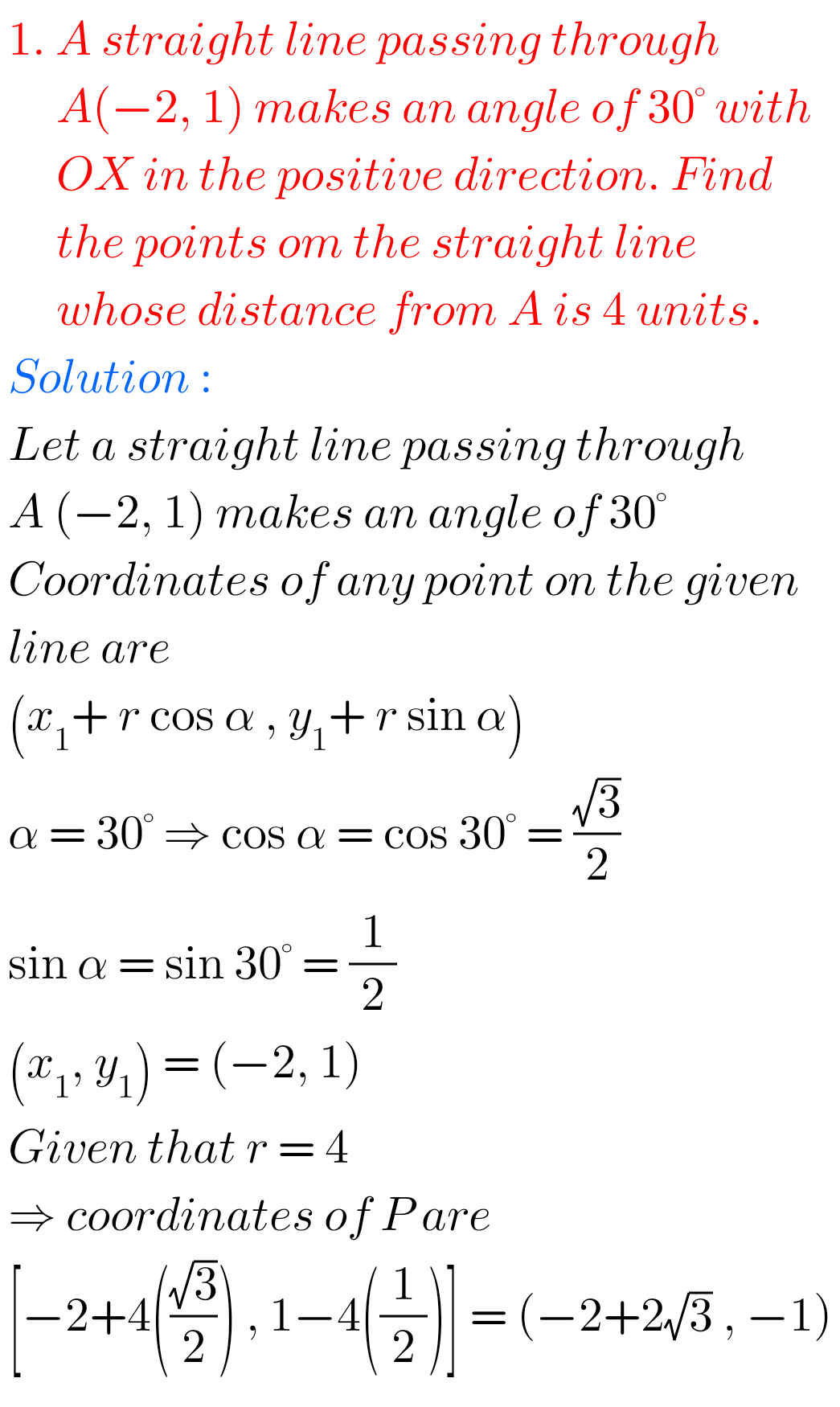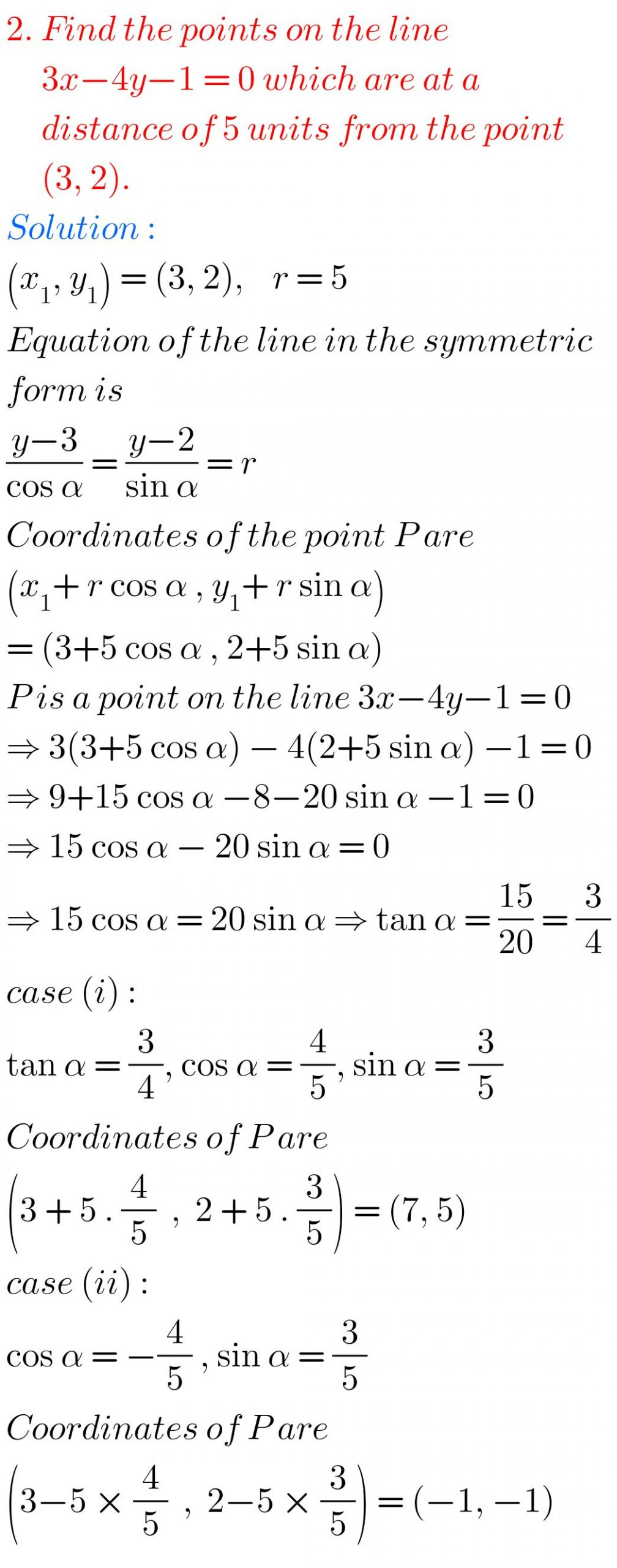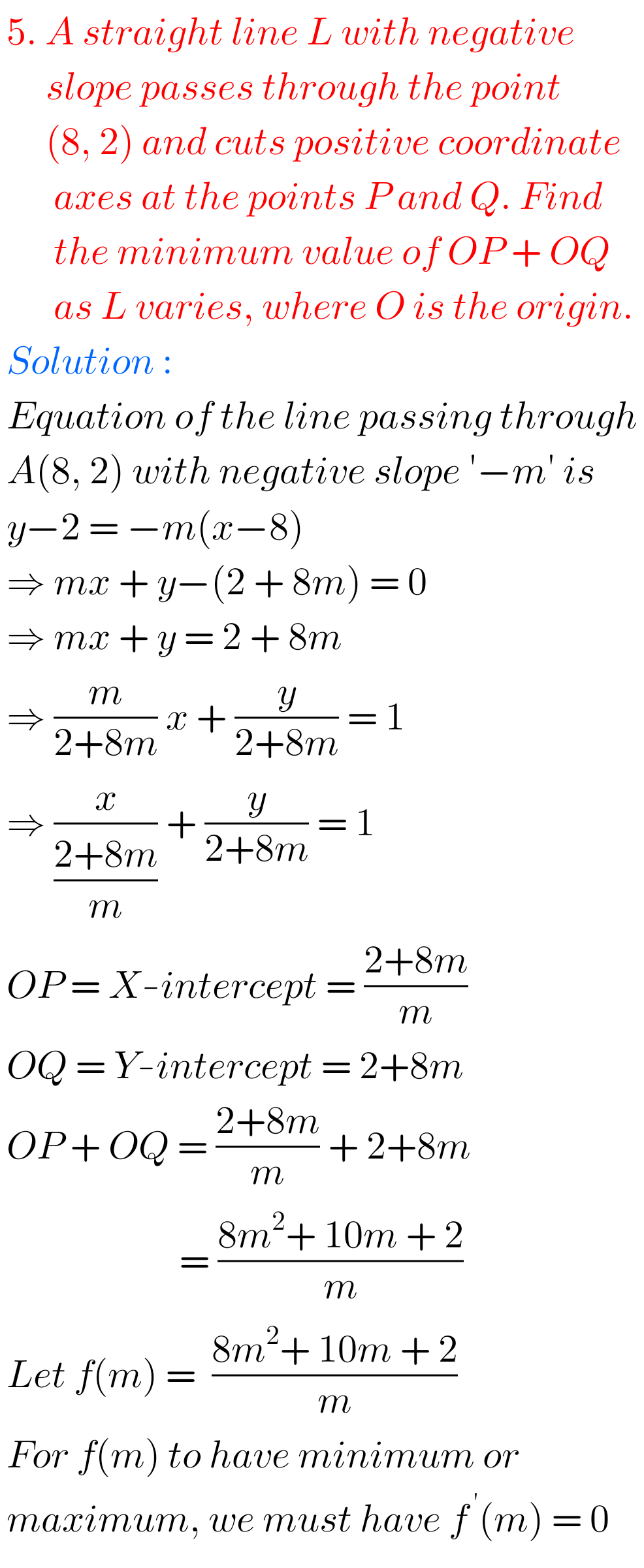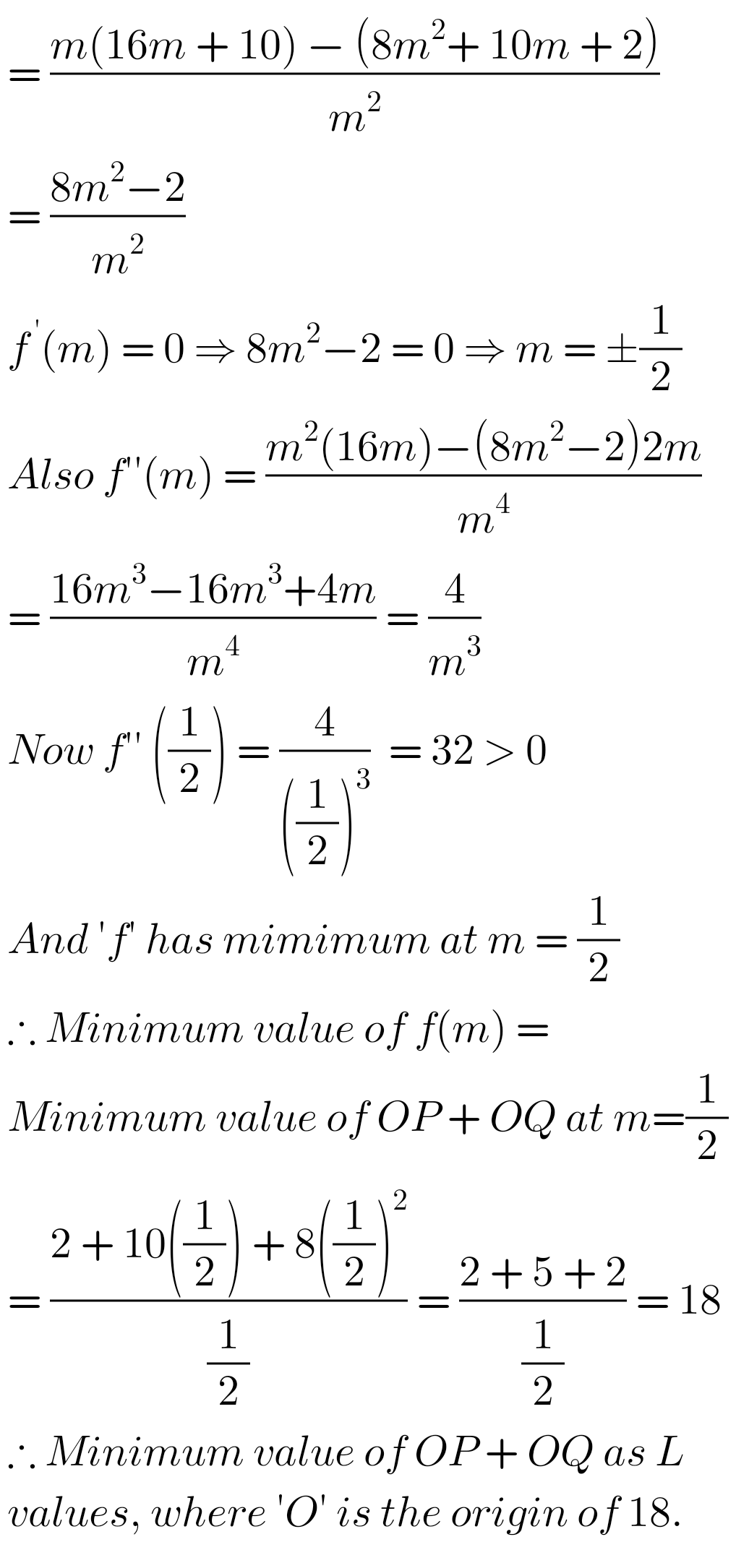Note: Observe the solutions and try them in your own method.

SSC Maths solutions for class 10

Inter Maths 1A textbook solutions

Ncert maths class 6 chapter 14 solutions

Nios maths 311 book 1 Sets 1.1 solutions